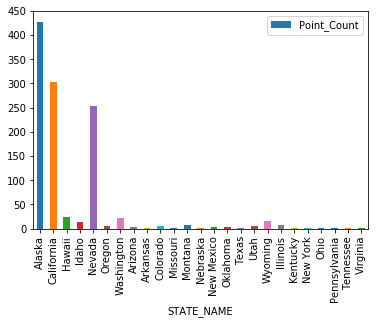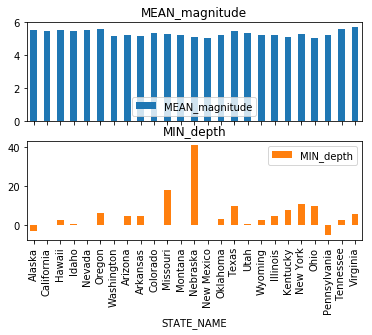ArcGIS Developer
Dashboard

# Summarize Data¶

The `features` module packs a set of data summarization tools to calculate total counts, lengths, areas, and basic descriptive statistics of features and their attributes within areas or near other features. You can access these tools using the `summarize_data` sub module.

## Aggregate points¶

In this example, let us observe how to use `aggregate_points` tool to summarize data from spot measurements by area. To learn more about this tool and the formula it uses, refer to the documentation here

In :
```# connect to GIS
from arcgis.gis import GIS
```
In :
```# search for USA states - area / polygon data
states_search = gis.content.search("title:'USA States'",
"feature layer", max_items=1)
states_item = states_search
states_item
```

Lets draw the layers on a map and observe how they are distributed

In [ ]:
```map1 = gis.map("USA")
map1
```In :
```map1.add_layer(states_item)
```
In :
```map1.add_layer(eq_item)
```

### Aggregate earthquakes by state¶

As you can see, a number of earthquakes fall on the boundary of tectonic plates (ring of fire). However, there are a few more dispersed into other states. It would be interesting to aggregate all the earthquakes by state and plot that as a chart.

The `aggregate_points` tool in the `summarize_data` sub module is a valid candidate for such analyses. The example below shows how to run this tool using ArcGIS API for Python.

To start with, let us access the layers in the states and earthquakes items and view their attribute information to understand how the data can be summarized

In :
```eq_fl = eq_item.layers
states_fl = states_item.layers
```

We have accessed the layers in the items as `FeatureLayer` objects. We can query the `fields` property to understand what kind of attribute data is stored in the layers

In :
```#query the fields in eq_fl layer
for field in eq_fl.properties.fields:
print(field['name'])
```
```objectid
datetime
latitude
longitude
depth
magnitude
magtype
nbstations
gap
distance
rms
source
eventid
occurrence_time
```
In :
```# similarly for states data
for field in states_fl.properties.fields:
print(field['name'], end="\t")
```
`FID	STATE_NAME	STATE_FIPS	SUB_REGION	STATE_ABBR	POPULATION	POP_SQMI	POP2010	POP10_SQMI	WHITE	BLACK	AMERI_ES	ASIAN	HAWN_PI	HISPANIC	OTHER	MULT_RACE	MALES	FEMALES	AGE_UNDER5	AGE_5_9	AGE_10_14	AGE_15_19	AGE_20_24	AGE_25_34	AGE_35_44	AGE_45_54	AGE_55_64	AGE_65_74	AGE_75_84	AGE_85_UP	MED_AGE	MED_AGE_M	MED_AGE_F	HOUSEHOLDS	AVE_HH_SZ	HSEHLD_1_M	HSEHLD_1_F	MARHH_CHD	MARHH_NO_C	MHH_CHILD	FHH_CHILD	FAMILIES	AVE_FAM_SZ	HSE_UNITS	VACANT	OWNER_OCC	RENTER_OCC	NO_FARMS12	AVE_SIZE12	CROP_ACR12	AVE_SALE12	SQMI	Shape__Area	Shape__Length	`

Let us aggreate the points by state and summarize the `magnitude` field and use `mean` as the summary type.

In :
```from arcgis.features import summarize_data
sum_fields = ['magnitude Mean', 'depth Min']
eq_summary = summarize_data.aggregate_points(point_layer = eq_fl,
polygon_layer = states_fl,
keep_boundaries_with_no_points=False,
summary_fields=sum_fields)
```

When running the tool above, we did not specify a name for the `output_name` parameter. Hence the analyses results were not stored on the portal, instead stored in the variable `eq_summary`.

In :
```eq_summary
```
Out:
`{'aggregated_layer': <FeatureCollection>, 'group_summary': <FeatureCollection>}`
In :
```# access the aggregation feature colleciton
eq_aggregate_fc = eq_summary['aggregated_layer']

#query this feature collection to get a data as a feature set
eq_aggregate_fset = eq_aggregate_fc.query()
```

`FeatureSet` objects support visualizing attribute information as a pandas dataframe. This is a neat feature since you do not have to iterate through each feature to view their attribute information.

Let us view the summary results as a pandas dataframe table. Note, the `aggregate_points` tool appends the polygon layer's original set of fields to the analysis result in order to provide it context

In :
```aggregation_df = eq_aggregate_fset.sdf
```
Out:
AGE_10_14 AGE_15_19 AGE_20_24 AGE_25_34 AGE_35_44 AGE_45_54 AGE_55_64 AGE_5_9 AGE_65_74 AGE_75_84 ... STATE_ABBR STATE_FIPS STATE_NAME SUB_REGION Shape_Area Shape_Length Shape__Area Shape__Length VACANT WHITE
0 50816 52141 54419 103125 92974 111026 85909 50887 35350 14877 ... AK 02 Alaska Pacific None None 8.101401e+12 5.924954e+07 48909 473576
1 2590930 2823940 2765949 5317877 5182710 5252371 4036493 2505839 2275336 1370210 ... CA 06 California Pacific None None 6.479799e+11 5.237952e+06 1102583 21453934
2 81539 85994 95829 185333 176373 193765 175562 83361 100523 64377 ... HI 15 Hawaii Pacific None None 1.888067e+10 1.370185e+06 64170 336599
3 116955 115359 108209 208965 191609 208537 180313 121195 109534 59892 ... ID 16 Idaho Mountain None None 4.235214e+11 3.858889e+06 88388 1396487
4 183173 182600 177509 387286 383043 376527 315499 183077 197781 96391 ... NV 32 Nevada Mountain None None 4.807101e+11 3.025639e+06 167564 1786688

5 rows × 62 columns

Thus, from our data, of the 50 states, only 23 have had earthquakes. Let us plot a bar chart to view which states had the most earthquakes

In :
```%matplotlib inline
aggregation_df.plot('STATE_NAME','Point_Count', kind='bar')
```
Out:
`<matplotlib.axes._subplots.AxesSubplot at 0x1e46225f2e8>`Clearly, California tops the list with the most number of earthquakes. Let us view what the average intensity and minimum depth is in the plots below:

In :
```aggregation_df.plot('STATE_NAME',['MEAN_magnitude', 'MIN_depth'],kind='bar', subplots=True)
```
Out:
```array([<matplotlib.axes._subplots.AxesSubplot object at 0x000001E46223C3C8>,
<matplotlib.axes._subplots.AxesSubplot object at 0x000001E46235B518>],
dtype=object)```Feedback on this topic?